# ISEE Upper Level Quantitative : How to find the length of the side of a triangle

## Example Questions

### Example Question #1 : How To Find The Length Of The Side Of A Triangle

Which of the following could be the lengths of the three sides of a scalene triangle?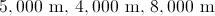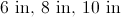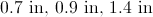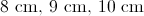All of the other choices are possible lengths of a scalene triangle

All of the other choices are possible lengths of a scalene triangle

Explanation:

A scalene triangle, by definition, has sides all of different lengths. Since all of the given choices fit that criterion, the correct choice is that all can be scalene.

### Example Question #2 : How To Find The Length Of The Side Of A Triangle

Given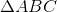with right angle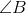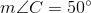Which is the greater quantity?

(a)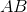(b)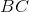(b) is greater

It is impossible to tell from the information given

(a) and (b) are equal

(a) is greater

(a) is greater

Explanation: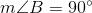The sum of the measures of the angles of a triangle is 180, so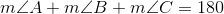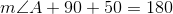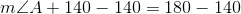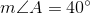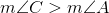, so the side opposite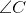, which is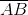, is longer than the side opposite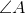, which is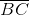. This makes (a) the greater quantity.

### Example Question #3 : How To Find The Length Of The Side Of A Triangle

Givenwith obtuse angle, which is the greater quantity?

(a)(b)(b) is greater.

(a) is greater.

(a) and (b) are equal.

It is impossible to tell from the information given.

(b) is greater.

Explanation:

To compare the lengths ofandfrom the angle measures, it is necessary to know which of their opposite angles -and, respectively - is the greater angle. Sinceis the obtuse angle, it has the greater measure, andis the longer side. This makes (b) greater.

### Example Question #4 : How To Find The Length Of The Side Of A Trianglehas obtuse angle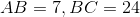. Which is the greater quantity?

(a)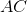(b)It is impossible to tell from the information given.

(a) is greater.

(b) is greater.

(a) and (b) are equal.

(a) is greater.

Explanation:

Sinceis the obtuse angle of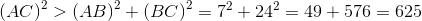.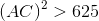,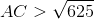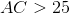,

so (a) is the greater quantity.

### Example Question #5 : How To Find The Length Of The Side Of A Triangle

Givenwith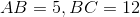. Which is the greater quantity?

(a)(b)(a) and (b) are equal.

(b) is greater.

(a) is greater.

It is impossible to tell from the information given.

(b) is greater.

Explanation:

Use the Triangle Inequality: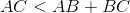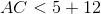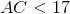This makes (b) the greater quantity.

### Example Question #6 : How To Find The Length Of The Side Of A Triangle

Givenwith. Which is the greater quantity?

(a)(b)(a) is greater.

(a) and (b) are equal.

It is impossible to tell from the information given.

(b) is greater.

It is impossible to tell from the information given.

Explanation:

By the Converse of the Pythagorean Theorem,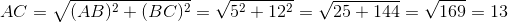if and only ifis a right angle.

However, ifis acute, then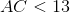;  ifis obtuse, then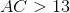.

Since we do not know whetheris acute, right, or obtuse, we cannot determine whether (a) or (b) is greater.

### Example Question #7 : How To Find The Length Of The Side Of A Triangleis acute;. Which is the greater quantity?

(a)(b)(a) is greater.

(b) is greater.

(a) and (b) are equal.

It is impossible to tell from the information given.

(b) is greater.

Explanation:

Sinceis an acute triangle,is an acute angle, and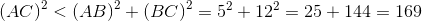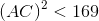,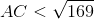(b) is the greater quantity.

### Example Question #8 : How To Find The Length Of The Side Of A Triangle

Given: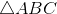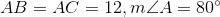. Which is the greater quantity?

(a) 18

(b)(b) is the greater quantity

(a) is the greater quantity

It is impossible to determine which is greater from the information given

(a) and (b) are equal

(a) is the greater quantity

Explanation:

Suppose there exists a second triangle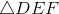such that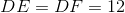and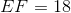. Whether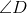, the angle opposite the longest side, is acute, right, or obtuse can be determined by comparing the sum of the squares of the lengths of the shortest sides to the square of the length of the longest: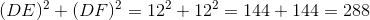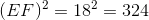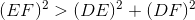, makingobtuse, so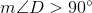.

We know thatand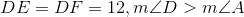.

Betweenand, we have two sets of congruent sides, with the included angle of the latter of greater measure than that of the former. It follows from the Side-Angle-Side Inequality (or Hinge) Theorem that between the third sides,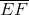is the longer. Therefore,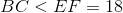.